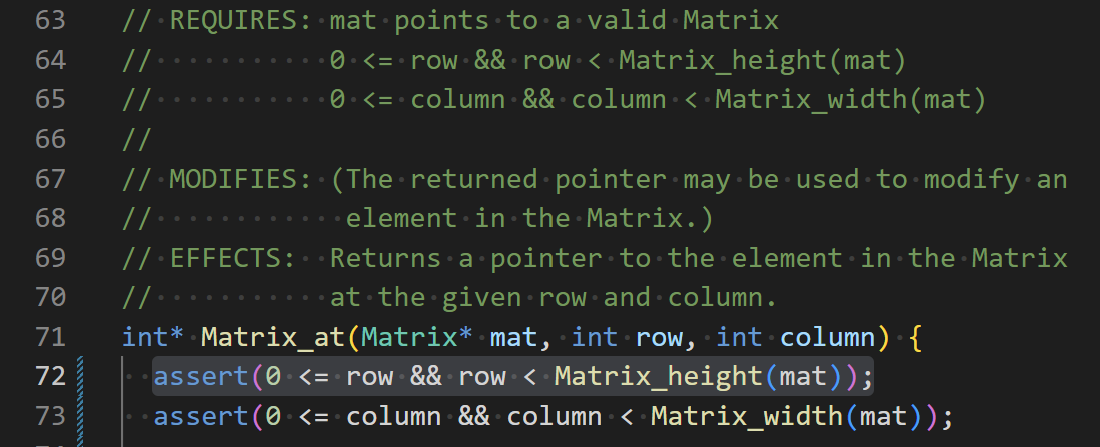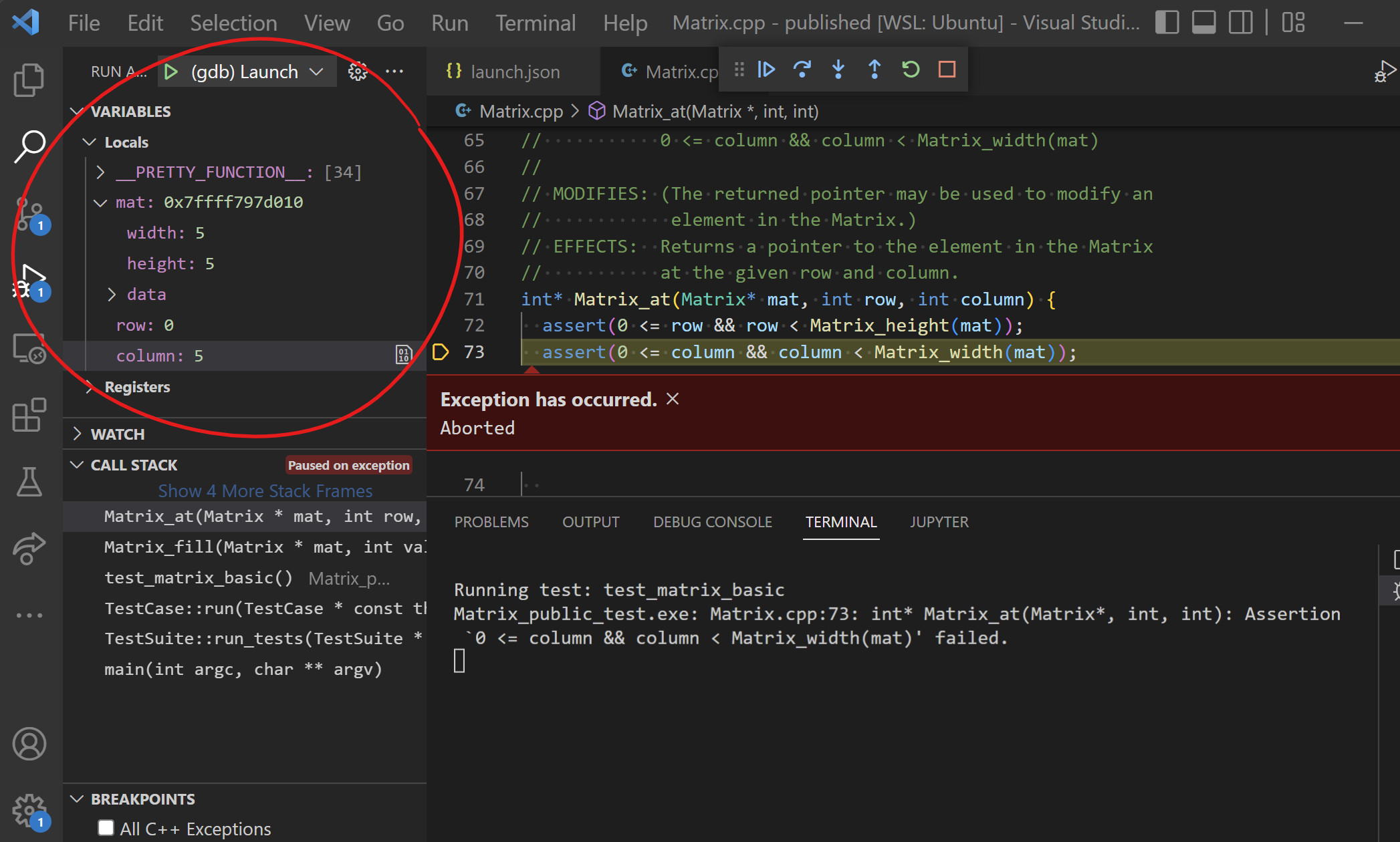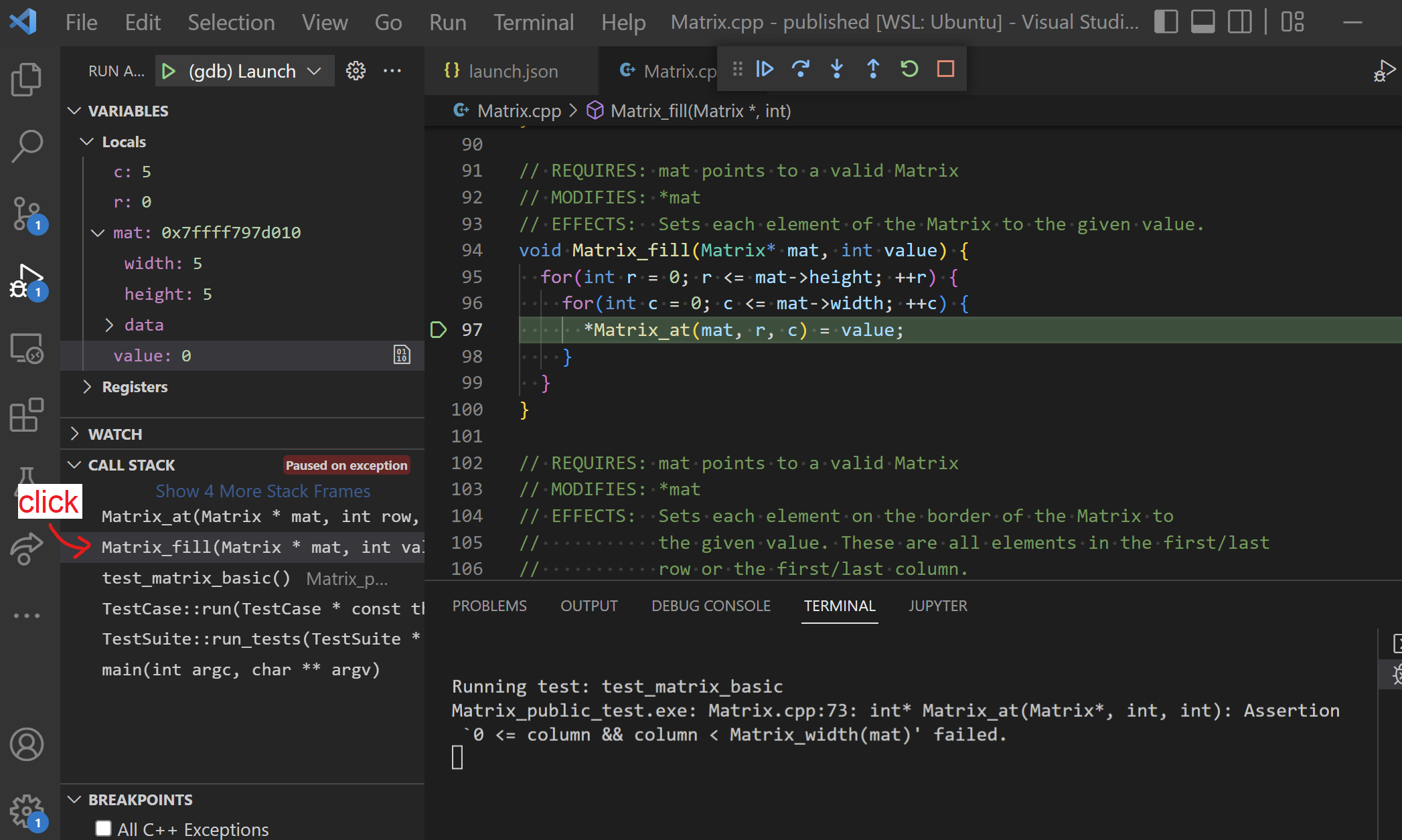# Assertions Guide

Assertions are a preemptive debugging tool. Add them to your code to detect bugs before they cause difficult-to-debug problems later in your code.

## Using Assertions

The `<cassert>` header defines an `assert()` macro that checks a boolean condition:

• If the condition is true, nothing happens.
• If the condition is false, the program crashes immediately.

For example:

``````// primer-spec-highlight-start
#include <cassert>
// primer-spec-highlight-end

int main() {
int x = 2;
int y = 5;
// primer-spec-highlight-start
assert(x + 3 == y); // true, all good :)
assert(x > y); // false, crash!! (╯°□°)╯︵ ┻━┻
// primer-spec-highlight-end
}
``````

We might see this output when running the above:

``````test.exe: test.cpp:7: int main(): Assertion `x > y' failed.
Aborted
``````

For debugging purposes, we use `assert()` to verify our program is behaving as intended and “fail fast” otherwise so that we can get to fixing the bug.

Assertions are intended for debugging, but you don’t have manually remove them before shipping production code. They can be turned off automatically by compiling with the `-DNDEBUG` flag.

### Sanity Check

You might run into situations in your code where you think “this could never happen” - and you’re often right, unless there’s a bug somewhere! Adding assertions to “prevent the impossible” will expose such bugs.

For example, you might find that certain paths through the code are unreachable if everything is working as expected, and you can encode this as an assertion. Here’s an example, pulled from the project 3 specification.

``````Player * Player_factory(const std::string &name,
const std::string &strategy) {
// We need to check the value of strategy and return
// the corresponding player type.
if (strategy == "Simple") {
// The "new" keyword dynamically allocates an object.
return new SimplePlayer(name);
}

// Repeat for each other type of Player
...

// primer-spec-highlight-start
// Assuming we've checked for each kind of Player
// above, the code should never get here. If we do...
// something is very wrong and the assert lets us know!
assert(false);
// primer-spec-highlight-end
}
``````

The `assert(false)` here is also helpful to suppress a warning the compiler might otherwise give because it’s worried our code could reach the end of the function without returning anything (g++ phrases this as “warning: control reaches end of non-void function”). But, with the assertion, the end of the function can literally never be reached and the compiler doesn’t give that warning.

Or, you might write code to ensure some property, like forcing a user to enter a non-negative number. You could add an assertion to verify the code has actually achieved that. Consider this example code:

``````int get_order_quantity() {
cout << "How many items would you like to order?" << endl;

// Get user input
int quantity;
cin >> quantity;

// Prompt them again if the input was invalid
if (quantity < 0) {
cout << "Invalid input. Please enter a non-negative number." << endl;
cin >> quantity;
}

// primer-spec-highlight-start
// We should have a valid quantity after the user interaction above.
// (Unless there's a bug somewhere - this will sanity check!)
assert(quantity >= 0);
// primer-spec-highlight-end
return quantity;
}
``````

That assertion proves worthwhile, since there is a bug in the code! Because the `if` statement only checks the first input for validity, an invalid negative input for the second `cin >> quantity` could sneak through. But, it would be immediately caught by the assertion and is easily debugged.

### Verify Preconditions

Use assertions to verify the `REQUIRES` clause for each function whenever possible. These are the preconditions that are required for the function to have a chance of working correctly, so verifying them up front is a good idea.

#### Example: `Matrix_at()` Preconditions

Here’s an example of assertions you could use in function that provides access to data at a given row/column within a `Matrix` struct:

``````// primer-spec-highlight-start
// REQUIRES: mat points to a valid Matrix
//           0 <= row && row < Matrix_height(mat)
//           0 <= column && column < Matrix_width(mat)
// primer-spec-highlight-end
// EFFECTS:  Returns a pointer to the element in
//           the Matrix at the given row and column.
int* Matrix_at(Matrix* mat, int row, int column) {
// primer-spec-highlight-start
assert(0 <= row && row < mat->height);
assert(0 <= column && column < mat->width);
// primer-spec-highlight-end
// Implementation
// ...
}
``````

If we were to call `Matrix_at()` with parameters that are outside the bounds of the `Matrix`, we get a failed `assert` right away and can debug the issue. These assertions are also invaluable while debugging other code that uses `Matrix_at()` - if the assertions aren’t failing, you can safely rule out any out-of-bounds bugs and focus your attention elsewhere.

Of course, you can’t assert every single thing in every `REQUIRES` clause. Some of them can’t possibly be checked. Here’s another example:

``````// REQUIRES: mat points to a valid Matrix
// primer-spec-highlight-start
//           the destination array is large enough
// primer-spec-highlight-end
// EFFECTS:  Copies all data from the matrix to
//           the given destination array
void copy_matrix_to_array(const Matrix* mat, int dest[]) {
// primer-spec-highlight-start
// Verify REQUIRES clause???
// primer-spec-highlight-end
// Implementation
// ...
}
``````

In this case, we can’t use an `assert` to verify that the destination array is large enough, because the `int[]` array parameter decays to an `int *` type that doesn’t contain information about the size of the original array.

### Check Invariants

The representation invariants of an abstract data type (ADT) express the conditions required for its present state to be valid. In other words, do the member variables of a given `struct` or `class` object make sense in terms of what it’s trying to represent? For example, Some examples:

• In a `Matrix` struct, the `width` and `height` members should be positive numbers
• In a Euchre `Card` class, the `suit` member must be `"spades"`, `"hearts"`, `"diamonds"`, or `"clubs"`

We try to write code carefully so that the representation invariants are set up correctly when an ADT object is created and preserved throughout any operations on it. And we’ll also rely on assuming they are true when we write code to work with the object. We can add assertions to verify those assumptions and fail fast if they’re ever broken.

#### Example: `Matrix` ADT

In a C-style ADT implemented with a `struct`, you can create a general helper to check invariants. For example, consider a `Matrix` ADT and a corresponding function to check its invariants:

``````struct Matrix {
int width;
int height;
int data[MAX_MATRIX_WIDTH * MAX_MATRIX_HEIGHT];
};

// EFFECTS: Uses assertions to verify the representation invariants
//          for the given Matrix.
void Matrix_check_invariants(const Matrix* mat) {
assert(mat); // Shouldn't be nullptr
assert(0 < mat->width && mat->width <= MAX_MATRIX_WIDTH);
assert(0 < mat->height && mat->height <= MAX_MATRIX_HEIGHT);
}
``````

Then, call it at as needed to proactively sanity check:

``````// REQUIRES: mat points to a valid Matrix
// EFFECTS:  Returns a pointer to the last element (at the
//           highest possible row/column) in the Matrix.
int * Matrix_last(Matrix* mat) {
// primer-spec-highlight-start
Matrix_check_invariants(mat);
// primer-spec-highlight-end
return &mat->data[mat->height * mat->width - 1];
}
``````

In the example above, you could think of this as a check on the REQUIRES clause that specifies `mat` must point to a valid `Matrix`. This keeps us from wasting time if, for example, `Matrix_last()` appears to be broken but the problem was really that we forgot to call `Matrix_init()` previously.

#### Example: `List` ADT

In a C++ style class, implement `check_invariants()` as a private member function. Call it after initializing member variables in the constructor and any time you make changes to your data representation (i.e. the member variables).

Here’s an example for a complex data structure called a linked list, in which individual nodes store data and connect to their neighbors via pointers:

``````class List {
private:
// Lists contain nodes, connected in a chain using pointers
struct Node {
Node *next;
Node *prev;
int datum;
};

// Member variables
Node *first;
Node *last;
int num_nodes;

// primer-spec-highlight-start
// Check represenation invariants with assert
void check_invariants() {
// Either it's empty with null first/last pointers,
// or it's not empty and neither should be null
assert(!first && !last || first && last);

// There's nothing before the first node
// and nothing after the last node.
assert(first->prev == nullptr);
assert(last->next == nullptr);

// Manually counting the number of nodes matches
// the number we're tracking in num_nodes
int count = 0;
for(Node *n = first; n; n = n->next) {
++count;
}
assert(count == num_nodes);
}
// primer-spec-highlight-end

public:

// Default constructor. Creates an empty list.
List : first(nullptr), last(nullptr), num_nodes(0) {
// primer-spec-highlight-start
check_invariants(); // ensure our list is set up
// primer-spec-highlight-end
}

//EFFECTS:  inserts datum into the front of the list
void push_front(const T &datum) {

// A bunch of complicated code that rearranges
// pointers to add a node to the list
// ...

// primer-spec-highlight-start
check_invariants(); // make sure we didn't screw up the pointers
// primer-spec-highlight-end
}
};
``````

## Debug Failed Assertions

Let’s say you’re running `Image_public_test.exe` on project 2 and one of your `Matrix_at()` assertions fails:

``````\$ ./Image_public_tests
Running test: test_image_basic
Image_public_test.exe: Matrix.cpp:72: int* Matrix_at(Matrix*, int, int): Assertion `0 <= row && row < Matrix_height(mat)' failed.
Aborted
``````

That’s nice, but just a line number (i.e. `Matrix.cpp:72`) doesn’t really give us much to go on:It should pause when the `assert` fails so that we can inspect the state of the program and figure out why the assertion is failing. First, check the local variables:It looks like the problem is that the `column` parameter is too large (given width 5, only 0-4 column indices are allowed). We can figure out where this is coming from by clicking on the the stack frame for the calling context, which is the `Matrix_fill()` function:Now we can actually see the call to `Matrix_at()` that broke its REQUIRES clause. In this implementation of `Matrix_fill()`, the `c` variable is allowed to reach value `5`, which is one too large. We need to adjust the conditions on the loops to fix this.

Because we had assertions in `Matrix_at()`, it warned us right away that `Matrix_fill()` was up to no good!

## Assertions for Testing

In this guide, we discuss assertions as a preemptive debugging tool. Assertions are also frequently used for unit testing, often in combination with a unit-testing framework such as the one we provide in EECS 280 that may provide additional constructs like `ASSERT_TRUE` or `ASSERT_EQUAL()`. We don’t address that context in this guide, and we don’t recommend mixing those special testing assertions into your code for debugging purposes.

## Acknowledgments

Original document written by James Juett jjuett@umich.edu.